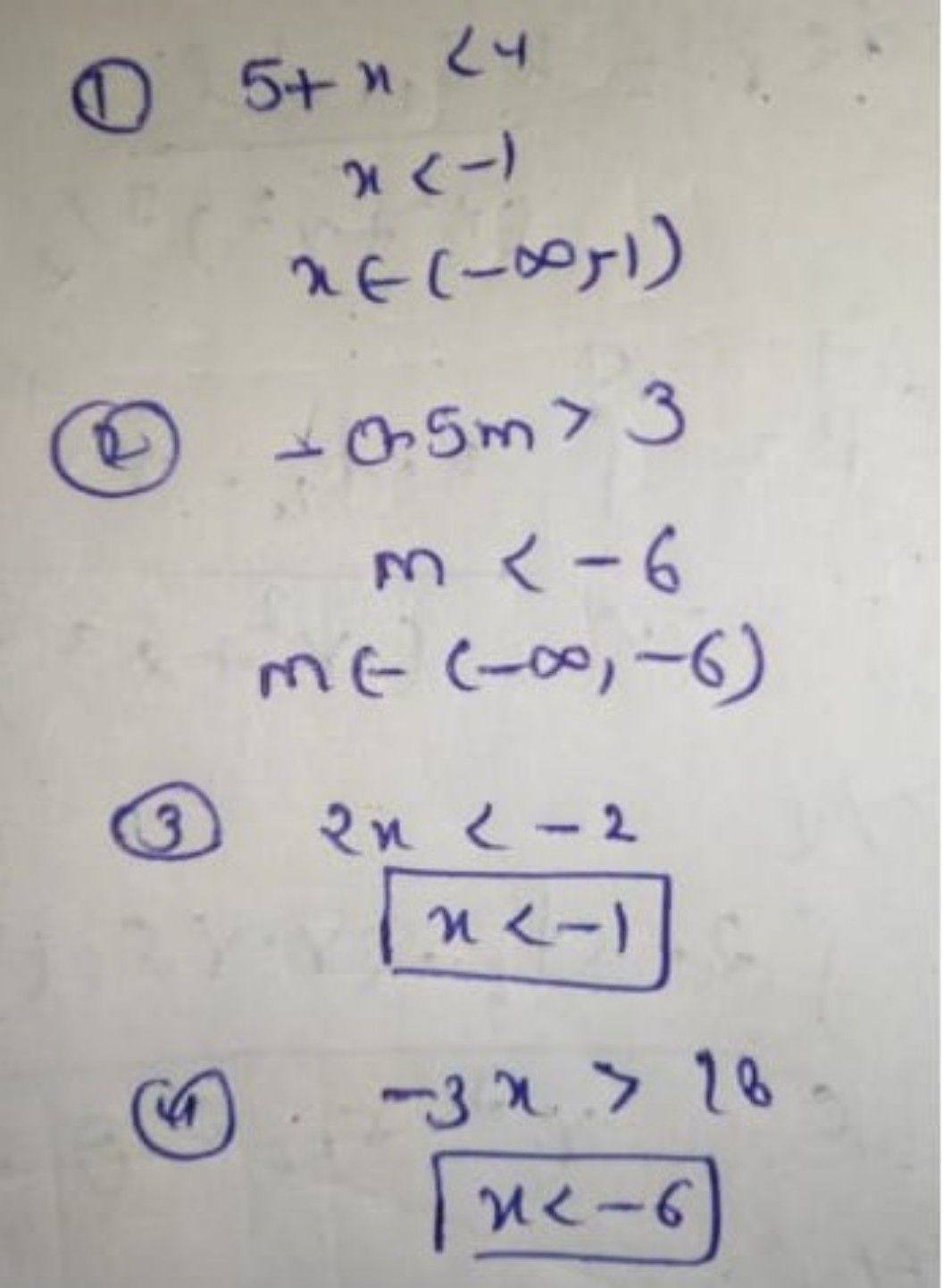Symbol
Problem$\bar{8}$ Find the values of x x that satisfy the inequality and plot the solution in interval notation $2$ on the number line. $\left(0\right)$ $5$ + $3$ $24$ b. $05n=26$ $4Q23°8\leq 0$ d. $7=8\right)x$ $-$ $25$ e. $5$ 14x + $5$ $5a%63$ $6=2346$ $853>2$ $3x=1$
10th-13th grade
Geometry
SolutionQanda teacher - lezlycrossplease do evaluate and please gift me coins